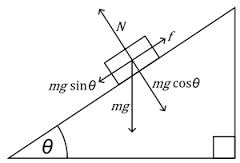# Friday, 13th June 2014This is a schematic diagram for the acceleration experiment I am working on.

Acceleration measured in this experiment is the sine component of ‘g’.

So now we can do this experiment by two methods.

1. By using motion sensor …. the data is recorded with uniform time intervals.
2. By using Photo-gates …… the time intervals are not uniform. time taken for traveling different distances can be measured and data can be used to calculate acceleration.